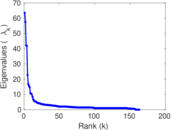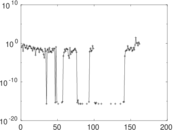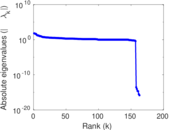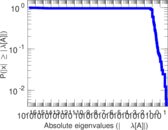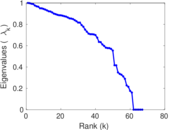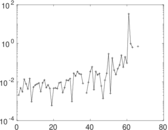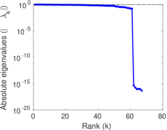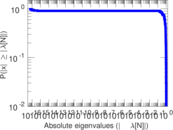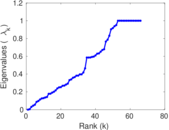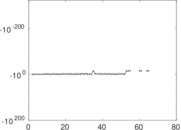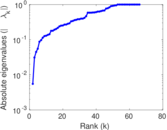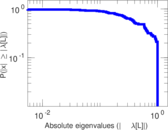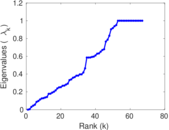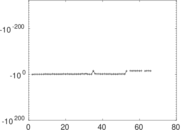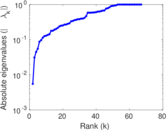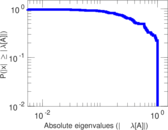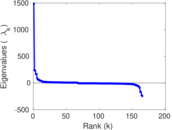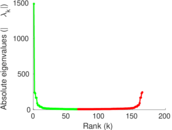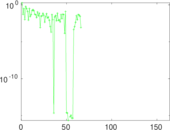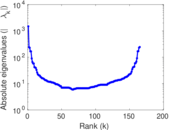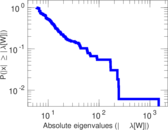# Wikibooks edits (sa)

This is the bipartite edit network of the Sanskrit Wikibooks. It contains users and pages from the Sanskrit Wikibooks, connected by edit events. Each edge represents an edit. The dataset includes the timestamp of each edit.

 Code `bsa` Internal name `edit-sawikibooks` Name Wikibooks edits (sa) Data source http://dumps.wikimedia.org/ AvailabilityDataset is available for download Consistency checkDataset passed all tests Category Authorship network Dataset timestamp 2017-10-20 Node meaning User, article Edge meaning Edit Network formatBipartite, undirected Edge typeUnweighted, multiple edges Temporal dataEdges are annotated with timestamps

## Statistics

 Size n = 1,493 Left size n1 = 165 Right size n2 = 1,328 Volume m = 1,956 Unique edge count m̿ = 1,223 Wedge count s = 153,628 Claw count z = 24,299,566 Cross count x = 3,055,754,961 Square count q = 327 4-Tour count T4 = 620,474 Maximum degree dmax = 742 Maximum left degree d1max = 742 Maximum right degree d2max = 59 Average degree d = 2.620 23 Average left degree d1 = 11.854 5 Average right degree d2 = 1.472 89 Fill p = 0.005 581 42 Average edge multiplicity m̃ = 1.599 35 Size of LCC N = 957 Diameter δ = 15 50-Percentile effective diameter δ0.5 = 3.572 61 90-Percentile effective diameter δ0.9 = 7.957 33 Median distance δM = 4 Mean distance δm = 4.826 69 Gini coefficient G = 0.649 525 Balanced inequality ratio P = 0.243 865 Left balanced inequality ratio P1 = 0.154 397 Right balanced inequality ratio P2 = 0.354 294 Relative edge distribution entropy Her = 0.788 597 Power law exponent γ = 6.945 85 Tail power law exponent γt = 2.981 00 Tail power law exponent with p γ3 = 2.981 00 p-value p = 0.001 000 00 Left tail power law exponent with p γ3,1 = 2.071 00 Left p-value p1 = 0.462 000 Right tail power law exponent with p γ3,2 = 3.781 00 Right p-value p2 = 0.248 000 Degree assortativity ρ = −0.237 089 Degree assortativity p-value pρ = 4.353 94 × 10−17 Spectral norm α = 63.520 4 Algebraic connectivity a = 0.005 510 41 Spectral separation |λ1[A] / λ2[A]| = 1.103 81 Controllability C = 927 Relative controllability Cr = 0.747 581

## Plots

### Fruchterman–Reingold graph drawing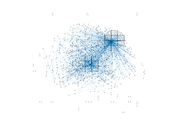### Degree distribution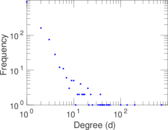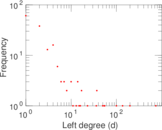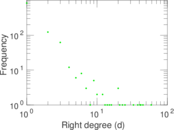### Cumulative degree distribution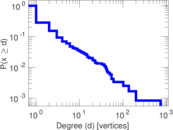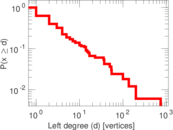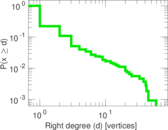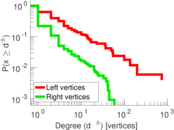### Lorenz curve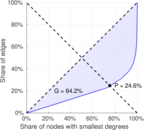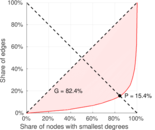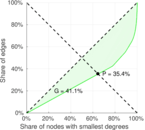### Spectral distribution of the adjacency matrix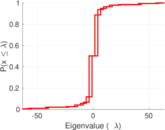### Spectral distribution of the normalized adjacency matrix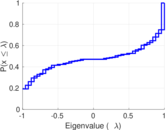### Spectral distribution of the Laplacian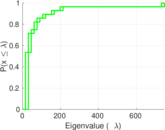### Spectral graph drawing based on the adjacency matrix### Spectral graph drawing based on the Laplacian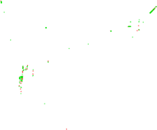### Spectral graph drawing based on the normalized adjacency matrix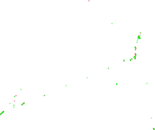### Degree assortativity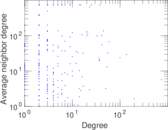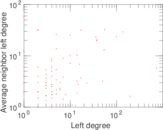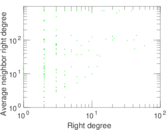### Zipf plot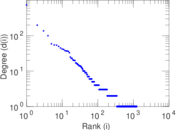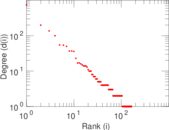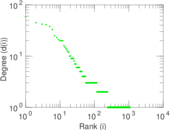### Hop distribution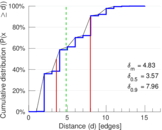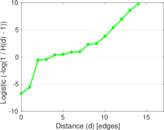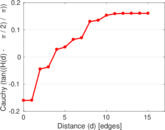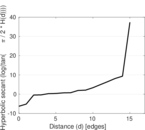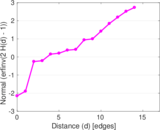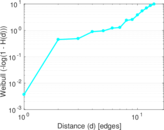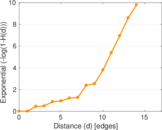### Double Laplacian graph drawing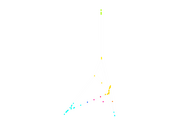### Delaunay graph drawing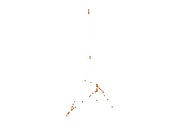### Edge weight/multiplicity distribution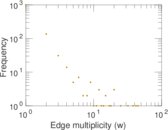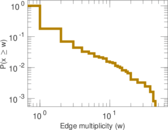### Temporal distribution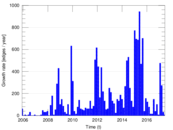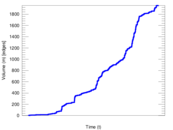### Temporal hop distribution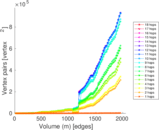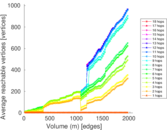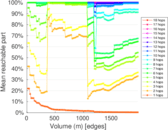### Diameter/density evolution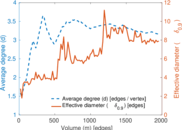### Matrix decompositions plots